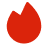# Nvidia计算机科学面试真题

BQ

Coding（1）
System Design（0）
Algorithm（11）
Ood（0）高频题（0）

Coding（1）
System Design（0）
Algorithm（11）
Ood（0）高频题（0）
1.LeetCode 128
2.LeetCode 227
3.LeetCode 1871
4.Find a unique integer in a list of duplicates
5.Fibonacci number
6.Pow
7.nth Fibonacci
8.Code review
9.最短路径
10.Tree Travesal
11.LeetCode 772
12.shortest distance to 1
1. LeetCode 128
Given an unsorted array of integers nums, return the length of the longest consecutive elements sequence.

You must write an algorithm that runs in O(n) time.

Example 1:

```Input: nums = [100,4,200,1,3,2]
Output: 4
Explanation: The longest consecutive elements sequence is [1, 2, 3, 4]. Therefore its length is 4.
```
Example 2:

```Input: nums = [0,3,7,2,5,8,4,6,0,1]
Output: 9
```

Constraints:

• 0 <= nums.length <= 105
• -109 <= nums[i] <= 109
2. LeetCode 227
Given a string s which represents an expression, evaluate this expression and return its value

The integer division should truncate toward zero.

You may assume that the given expression is always valid. All intermediate results will be in the range of [-231, 231 - 1].

Note: You are not allowed to use any built-in function which evaluates strings as mathematical expressions, such as eval().

Example 1:

```Input: s = "3+2*2"
Output: 7
```
Example 2:

```Input: s = " 3/2 "
Output: 1
```
Example 3:

```Input: s = " 3+5 / 2 "
Output: 5
```

Constraints:

• 1 <= s.length <= 3 * 105
• s consists of integers and operators ('+', '-', '*', '/') separated by some number of spaces.
• s represents a valid expression.
• All the integers in the expression are non-negative integers in the range [0, 231 - 1].
• The answer is guaranteed to fit in a 32-bit integer.
3. LeetCode 1871
You are given a 0-indexed binary string s and two integers minJump and maxJump. In the beginning, you are standing at index 0, which is equal to '0'. You can move from index i to index j if the following conditions are fulfilled:

• i + minJump <= j <= min(i + maxJump, s.length - 1), and
• s[j] == '0'.
Return true if you can reach index s.length - 1 in s, or false otherwise.

Example 1:

```Input: s = "011010", minJump = 2, maxJump = 3
Output: true
Explanation:
In the first step, move from index 0 to index 3.
In the second step, move from index 3 to index 5.
```
Example 2:

```Input: s = "01101110", minJump = 2, maxJump = 3
Output: false
```

Constraints:

• 2 <= s.length <= 105
• s[i] is either '0' or '1'.
• s == '0'
• 1 <= minJump <= maxJump < s.length
4. Find a unique integer in a list of duplicates
Given a list of integers where each integer, except one, appears twice. Find the one integer that only appears once.

5. Fibonacci number

6. Pow
Im‍‍‌‌‌‌‍‍‍‍‍‌‌‌‌‍‍‌‍‌plement a function that computes pow() in O(logN) time
7. nth Fibonacci
Implement a function that computes the nth Fibonacci element in O(logN) time
8. Code review
What is the output of the following code?

int main()

{

int a, b, c;

a = 9;

c = a + 1 + 1 * 0;

b = c++;

printf("a: %d, b: %d, c: %d\n", a, b, c);

return 0;

}
9. 最短路径
Find a shortest vertical path from the first row to bottom row in a 2D matrix

DP解决
10. Tree Travesal
Level order traversal

BST解决
11. LeetCode 772
Implement a basic calculator to evaluate a simple expression string.

The expression string contains only non-negative integers, '+', '-', '*', '/' operators, and open '(' and closing parentheses ')'. The integer division should truncate toward zero.

You may assume that the given expression is always valid. All intermediate results will be in the range of [-231, 231 - 1].

Note: You are not allowed to use any built-in function which evaluates strings as mathematical expressions, such as eval().

Example 1:

Input: s = "1+1"
Output: 2

Example 2:

Input: s = "6-4/2"
Output: 4

Example 3:

Input: s = "2*(5+5*2)/3+(6/2+8)"
Output: 21

Constraints:

1 <= s <= 104

s consists of digits, '+', '-', '*', '/', '(', and ')'.

s is a valid expression.
12. shortest distance to 1
"给一个input array eg：［1，0，0，1，0，1，0，0，1]
output [0，1，1，０，1，０，1，1，０]